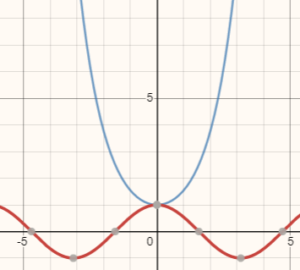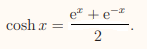# Hyperbolic Functions

Hyperbolic functions are a special class of transcendental functions, similar to trigonometric functions or the natural exponential function, ex. Although not as common as their trig counterparts, the hyperbolics are useful for some applications, like modeling the shape of a power line hanging between two poles.

Each trigonometric function has a corresponding hyperbolic function, with an extra letter “h”. For example, sinh(x), the Hhperbolic cosine function cosh(x) and tanh(x). While the “ordinary” trig functions parameterize (model) a curve, the hyperbolics model a hyperbola—hence the name.Graph of y = cosh(x) in blue and cos(x) in red. Cosh(x) has an absolute minimum at x = 0; It does not have an inflection point.
.

## Defining Using e(x)

Unlike their trigonometric counterparts, hyperbolic functions are defined in terms of the exponential function ex. For example, f(x) = cosh(x) is defined by:And sinh(x) is defined as:All of the remaining hyperbolic functions (see list below) can be defined in terms of these two definitions.

## Properties of Hyperbolic Functions

Hyperbolic functions can be even or odd functions.

• Even functions (symmetric about the y-axis): cosh(x) and sech(x),
• Odd functions (symmetric about the origin): All other hyperbolic functions are odd.

Some of these functions are defined for all reals: sinh(x), cosh(x), tanh(x) and sech(x). Two others, coth(x) and csch(x) are undefined at x = 0 because of a vertical asymptote at x = 0.

## Derivatives of Hyperbolic Functions

The derivatives of hyperbolic functions are almost identical to their trigonometric counterparts:

• sinh(x) = cosh(x)
• cosh(x) = sinh(x)
• tanh(x) = sech2(x)
• coth(x) = csch2(x)
• csch(x) = -csch(x) coth(x)
• sech(x) = sech(x) tanh(x)

## Limits

For x→ ∞, the limits of the hyperbolic functions are:

• limx → ± ∞ sinh(x) = ± ∞
• limx → ± ∞ cosh(x) = ∞
• limx → ± ∞ tanh(x) = ± 1
• limx → ± ∞ coth(x) = 0
• limx → ± ∞ csch(x) = 0
• limx → ± ∞ sech(x) = ± 1

## References

Graph of cosh(x): Desmos calculator.
Stewart. Math 133. Hyperbolic Functions. Retrieved November 24, 2019 from: https://users.math.msu.edu/users/magyar/Math133/6.7-Hyperbolic-Fcns.pdf

CITE THIS AS:
Stephanie Glen. "Hyperbolic Functions" From StatisticsHowTo.com: Elementary Statistics for the rest of us! https://www.statisticshowto.com/hyperbolic-functions/